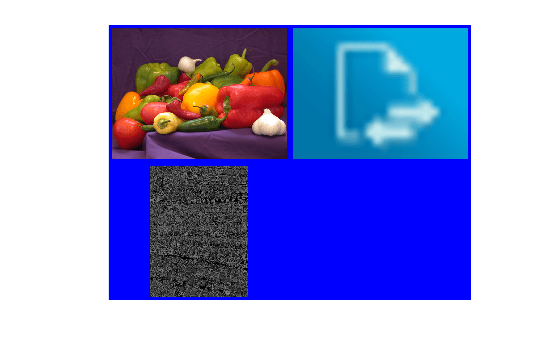# Concatenate images without image toolbox/montage function

13 views (last 30 days)
matquest on 7 Apr 2020
Commented: matquest on 9 Apr 2020
I am trying to "concatenate" some image files in a manner similar to either the montage or imtile functions, however, I need to be able to do this in vanilla matlab r2018a. The resulting image does not need to be displayed; it only needs to be created. I found this post How can I create a montage of images without using the montage function and I tweaked it to the following:
img3 = [img1 img2];
imshow(img3);
saveas(gcf, 'concatimg.png');
However, I have two problems which I am not sure how to remedy:
1. How can I concatenate images of arbitrary size? E.g., if img1 is 300x300 and img2 is 250x325 is there some function to just add some "buffer" pixels or do I need to manually resize the images (or should I just be using subplot)? Also, how can I add buffer pixels surrounding my images as in this example (taken from the imtile documentation):2. How can I save the composite image without the imshow and saveas function calls?

Geoff Hayes on 8 Apr 2020
Edited: Geoff Hayes on 8 Apr 2020
matquest - a very simple (hopefully accurate!) way would be to detemine the maximum width (columns) and height (rows) of the two images and then pad both so that they fill the larger image size. For example, padding the 300x300 image would result in a 300x325 image, and padding the 250x325 image would result in a 300x325 image too. Note that you don't have to pad to both dimensions just to the dimension that you want to concatenate across (so if you want to contcatenate the two images so that they are side by side, then both need to have the same number of rows. In this case, you would need 300x300 and 300x325 images where the first one maintains its size and the second is padded. Some code to pad in both dimensions might be
close all;
% sample images
img1 = uint8(zeros(300,300,3));
img1(:,:,1) = 255;
img2 = uint8(zeros(250,325,3));
img2(:,:,3) = 255;
maxNumRows = max(size(img1,1),size(img2,1));
maxNumCols = max(size(img1,2),size(img2,2));
numChannels = 1;
if ndims(img) == 3
numChannels = size(img,3);
end
% create padded image and place original in top left corner
% can modify this code if you want the padding to appear elsewhere
You can use imwrite to save the composite image to file.
matquest on 9 Apr 2020
Exactly what I meant! Thank you; it works perfectly.

R2018a

### Community Treasure Hunt

Find the treasures in MATLAB Central and discover how the community can help you!

Start Hunting!